# The Binary Partition of Integers

"Primary partitions define the integer and are symbolised by a broken horizontal line:".

"Secondary partitions define the partition structure of an integer and are symbolised by a solid horizontal line:".

Unlike material space which is granular, numerical space is infinitely divisible. Therefore because the integer 1 is infinitely divisible it is an infinity. The integer 1 is a simple infinity.

Because the integer 1 is "simple" it may best be symbolised as an undivided line:and because it is infinite a more illuminating symbol would be this: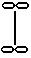-that is an undivided line with an infinity symbol at each end.

To define a particular number n from infinity the infinite line must be divided by n-1 partitions. For example 4 is defined from the infinite line by 4-1=3 partitions thus:ieEach of the 4 segments are equal, that is they are all infinite (ie simple undivided infinities identical with the integer 1) so we can see that integer 4 itself is also infinite. In fact all numbers are infinite.

It is understood that in every case the infinite line is the basis of quantity so for short hand thecan be left out: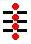Further, with understanding, each segment can be seen merely by the "spaces" surrounding the 3 partitions so integer 4 can be written thus: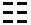Without altering the primary partition structure (which defines the infinity as the integer 4 ie 4 distinct integer 1's) how many ways can integer 4 be divided (into smaller integers)?

To illuminate the inherent pattern underlying the division of integers into smaller integers we employ secondary partitions.

4 may be partitioned 5 ways thus:
 1+1+1+1 2+1+1 2+2 3+1 4

Note that some of the partitions can have different orders thus:
 1+1+1+1 - - =1 Pemutation 2+1+1 1+2+1 1+1+2 =3 Pemutations 2+2 - - =1 Pemutation 3+1 1+3 - =2 Pemutations 4 - - =1 Pemutation - - - =8 Total

A secondary partition overlays a primary partition. For example:= integer 4 in an unpartitioned form.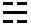=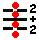=2+2=4.

In other words the primary partitions define where the integer can be split into smaller integers by the secondary partitions.

There are 8 permutations of the primary and secondary partitions over 3 places(ie for integer 4):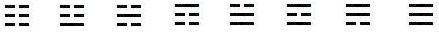Each of the 8 binary permutations corresponds exactly with a partition pattern:(reading the binary figures from top to bottom)

All mathematical structures are binary partition structures. All mathematical operations are changes in binary partition structure.

Primary partitions define the integer and are symbolised by a broken horizontal line:.

Secondary partitions define the partition structure of an integer and are symbolised by a solid horizontal line:.

The binary partition patterns for all integers and their interplay form a perfect unchanging pattern. Pascal's Triangle(or Mount Meru as it was known long before Pascal) is the foundation of this pattern: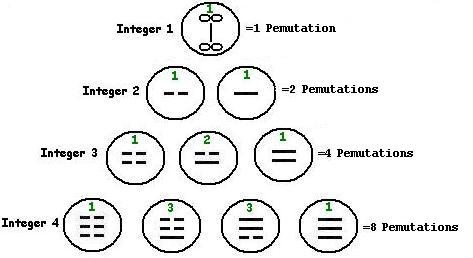The pattern for the first four integers. This pattern expands infinitely. The green numbers correspond with the traditional Pascal's Triangle pattern. Each level of the triangle also corresponds with an n-dimensional simplex.

The 8 permutations of the binary partitions for integer 4 correspond exactly with the 8 vertices of the cube. The 12 edges of the cube represent a simple transition from one partition line into the other. The 6 faces correspond with the transition of two partition lines into the other. The 1 center corresponds with all three partition lines changing. These 12+6+1 =the 19 possible transitions for the 8 integer 4 partition patterns.

the 8+the 19=27. For every integer the number of permutations is a power of 2 and can be expressed with binary figures. For every integer these permutations + their transitions is a power of 3 and can be expressed with trinary figures.

The partition patterns and the transitions for every integer correspond with a particular n-dimensional hyper-cube. This earlier (ie needs some updating )page helps illustrate this:
Hyper Cubes and CombinatoricsSearch on Amazon:
philosophy or...?